# 弧长

（请先去阅读关于 导数积分 的内容）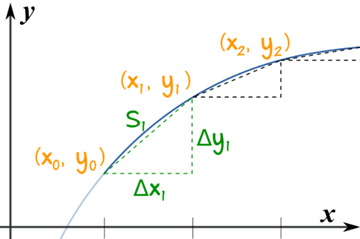x0x1

S1 = (x1 − x0)² + (y1 − y0

S1 = (Δx1)² + (Δy1

S2 = (Δx2)² + (Δy2
S3 = (Δx3)² + (Δy3
……
……
Sn = (Δxn)² + (Δyn

S ≈
n
Σ
i=1
(Δxi)² + (Δyi

• 让所有的 Δxi 都是 一样长，那么我们便可以把它们从平方根里拿出来，
• 把总和变成一个积分。

S ≈
n
Σ
i=1
(Δxi)² + (Δxi)²(Δyi/Δxi

S ≈
n
Σ
i=1
(Δxi)²(1 + (Δyi/Δxi)²)

(Δxi 从平方根里拿出来：

S ≈
n
Σ
i=1
1 + (Δyi/Δxi  Δxi

n 趋向 无穷大时 （趋向无穷多的线段，每段越来越小），总和变成：

S =
lim
n→∞
n
Σ
i=1
1 + (Δyi/Δxi  Δxi

S =
b
a
1 + (dy/dx dx

dy/dx 是函数 f(x) 的 导数，可以写成 f’(x)

S =
b
a
1 + (f’(x) dx

S =
d
c
1 + (g’(y) dy

• f’(x) 的 导数
• 1 + (f’(x))² dx 的 积分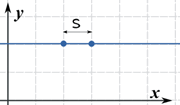### 例子：求 f(x) = 2 在 x=2 和 x=3 之间的距离

f(x) 是条水平线，所以导数是 f’(x) = 0

 开始： S =     3 ∫   2 √1 + (f’(x))² dx 代入 f’(x) = 0： S =     3 ∫   2 √1 + (0)² dx 简化： S =     3 ∫   2 dx 求积分： S = (3-2) = 1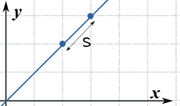### 例子：求f(x) = x 在 x=2 和 x=3 之间的长度

 开始：: S =     3 ∫   2 √1 + (f’(x))² dx 代入 f’(x) = 1：: S =     3 ∫   2 √1 + (1)² dx 简化： S =     3 ∫   2 √2 dx 再简化： S = √2     3 ∫   2 dx 求积分： S = √2(3-2) = √2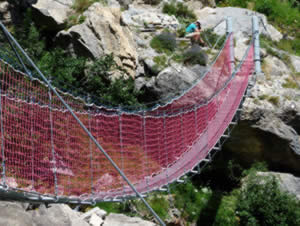### 例子：在峡谷上空每隔6米放一根金属杆。 求形状如以下曲线的索桥的长度：

f(x) = 5 cosh(x/5)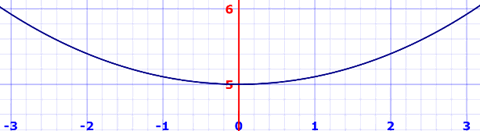f(x) = a cosh(x/a)

a 的值越大，线中间下垂越小
"cosh" 是 双曲余弦 函数。

 开始： S =     b ∫   0 √1 + (f’(x))² dx 代入 f’(x) = sinh(x/a)： S =     b ∫   0 √1 + sinh²(x/a) dx 用这个恒等式   1 + sinh²(x/a) = cosh²(x/a)： S =     b ∫   0 √cosh²(x/a) dx 简化： S =     b ∫   0 cosh(x/a) dx 求积分： S = a sinh(b/a)

S = 2a sinh(b/a)

S = 2×5 sinh(3/5)
= 6.367米
（精确到最近的毫米）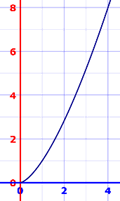### 例子：求 y = x(3/2) 在 x = 0 到 x = 4 之间的长度

 开始： S =     4 ∫   0 √1 + (f’(x))² dx 代入 (3/2)x(1/2)： S =     4 ∫   0 √1 + ((3/2)x(1/2))² dx 简化： S =     4 ∫   0 √1 + (9/4)x dx

• u = 1 + (9/4)x
• du = (9/4)dx
• (4/9)du = dx
• 上下限：u(0)=1 和 u(4)=10
 得到： S =     10 ∫   1 (4/9)√u du 求积分： S = (8/27) u(3/2) 从 1 到 10 计算： S = (8/27) (10(3/2) - 1(3/2)) = 9.073……

S =
b
a
1 + (f’(x) dx

• 取 f(x) 的导数
• 写下弧长公式
• 简化并解积分